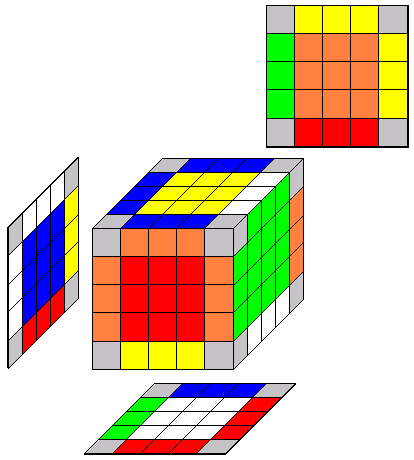# Solving the 5x5x5

back to Rubik's Cube

 8.2 Solve the edge-centers 8.2.1a Edge-Center at Front 8.2.1b(R' r') F (R r)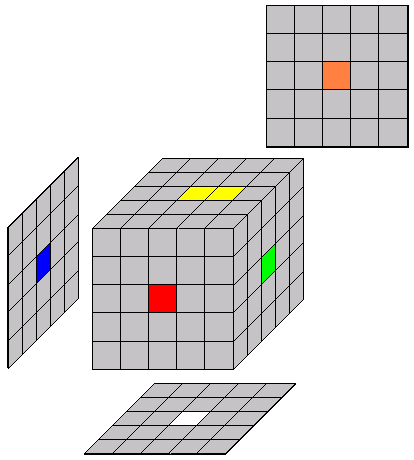8.2.2a Edge-Center on Bottom 8.2.2b(R2 r2) D (R2 r2)8.3 Solve the corner-centers 8.3.1a Corner-center at front 8.3.1b(R r) U (R' r') U (R r) U2 (R' r')8.3.2a Corner-center on bottom 8.3.2b(R2 r2) U (R2 r2) U (R2 r2) U2 (R2 r2)8.4 Solve the first four tredges - other wing edge 8.4.1a Wing edge is in the top layer on the left 8.4.1b Place at bFL (goto 8.5)R U' R' (D' d')8.4.2a Wing edge is in the top layer on the right 8.4.2b Place at bFL (goto 8.5)U' F' U F (D' d')8.4.3a Wing edge is in the bottom layer on the left 8.4.3b Place at bFL (goto 8.5)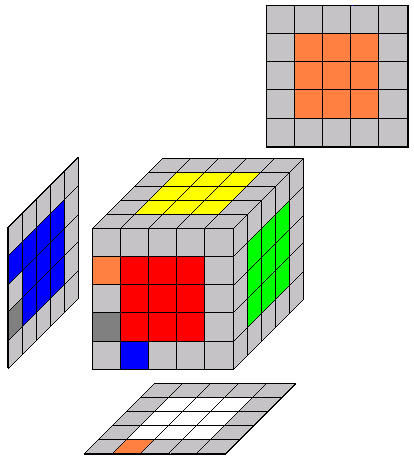D F D' F' (D' d')8.4.4a Wing edge is in the bottom layer on the right 8.4.4b Place at bFL (goto 8.5)R' D R (D' d')8.4.5a Wing edge is in a middle layer 8.4.5b Put it into the top layer (goto 8.4.1a)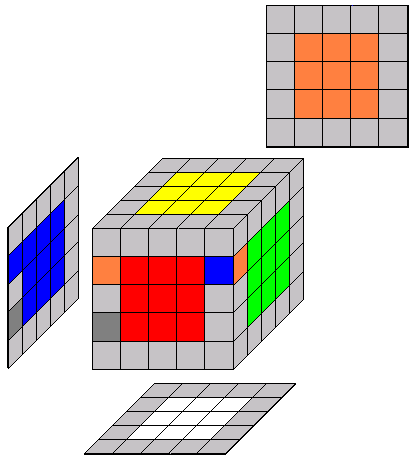R U' R'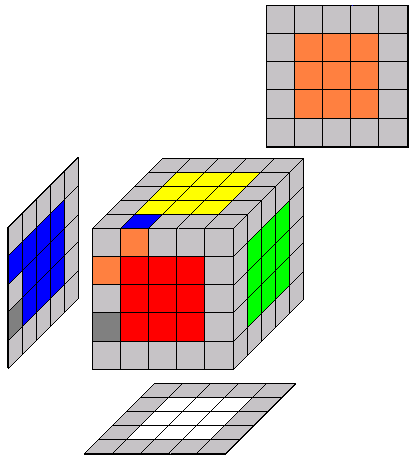8.5 Solve the first four tredges - center edge 8.5.1a Center edge is in the top layer and colors on front face are nonmatchingU' F' U F (U' u') (D d)8.5.2a Center edge is in the top layer and colors on front face are matchingR U' R' (U' u') (D d)8.5.3a Center edge is in the bottom layer and colors on front face are nonmatchingD F D' F' (U' u') (D d)8.5.4a Center edge is in the bottom layer and colors on front face are matchingR' D R (U' u') (D d)8.5.5a Wing edge is in a middle layer Put it into top layer and proceed with one of the "Center Edge is in the top layer" stepsR U' R'8.6 Solve the First Four Tredges - Store the edge 8.6.1a Move the completed tredge to the top layer and an uncompleted edge at the FT position to the middle.R U' R'8.7 The [flip] maneuver 8.7.1a This move flips the tredge at the FR position and shuffles some corners and tredges in U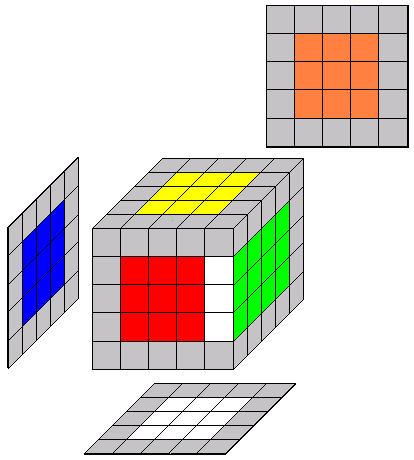R U R' F  R' F' R8.8 Solve the final four tredges - position other wing in FR First find a center edge in an unsolved middle tredge and rotate the cube so that this tredge is at FL. 8.8.1a A wing edge is in the FL position.  If the colors don't match, then Ignore this wing edge and find the other.  If both wing edges are in FL, then proceed to "8.8.5a flip adjacent wings".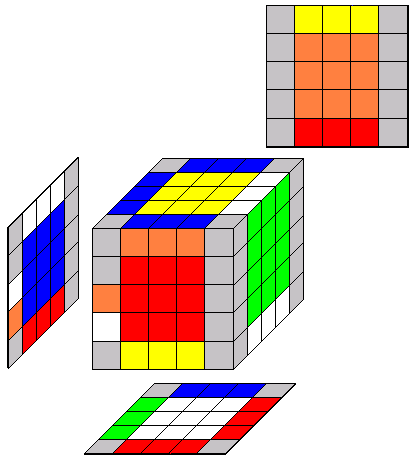8.8.2a A wing edge is already positioned in FR - proceed to 8.9.8.8.3a A wing edge is positioned at BR Move to FR (goto 8.9)R28.8.4a A wing edge is positioned at BL Move to FR (goto 8.9)B2 R28.8.5a Both wings are at FL but the colors don't match. Flip adjacent wings (goto 8.8)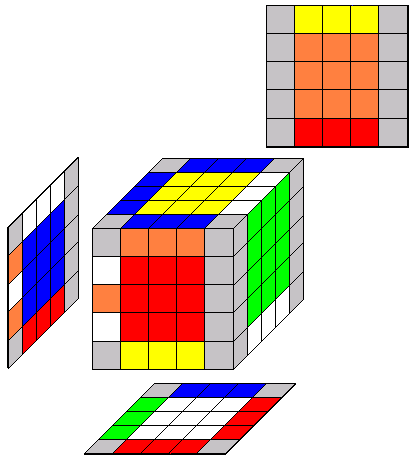(U' u') (D d) [flip] (U u) (D' d') flip =[R U R' F  R' F' R]8.9 Solve the final four tredges - move wing from FR to FL 8.9.1a A wing edge is dFR and the colors match.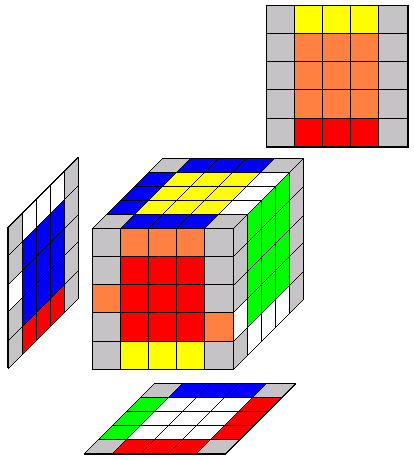(U' u') [flip] (U u) flip =[R U R' F  R' F' R]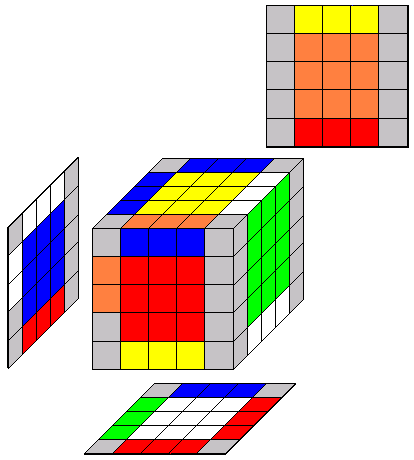8.9.2a A wing edge is uFR and the colors match.(D d) [flip] (D' d') flip =[R U R' F  R' F' R]8.9.3a A wing edge is dFR and the colors don't match.[flip] (D d) [flip] (D' d')flip =[R U R' F  R' F' R]8.9.4a A wing edge is uFR and the colors don't match.[flip] (U' u') [flip] (U u)flip =[R U R' F  R' F' R]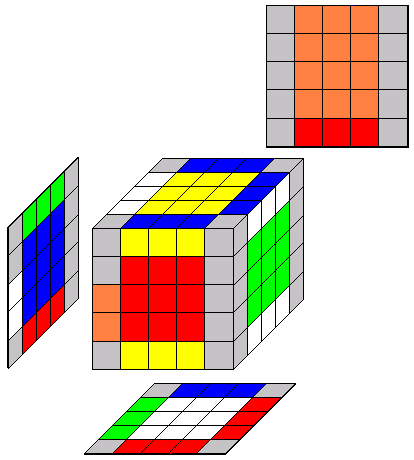8.9.5a Both wings are at FL but the colors don't match. Flip adjacent wings.(U' u') (D d) [flip] (U u) (D' d')flip =[R U R' F  R' F' R]8.9.11 Solve the final tredge 8.9.11a Half the time, the last tredge won't be solved with the eleventh tredge but two wing pieces will be flipped.r2 B2 U2 l U2 r' U2 r U2 F2 r F2  l' B2 r2